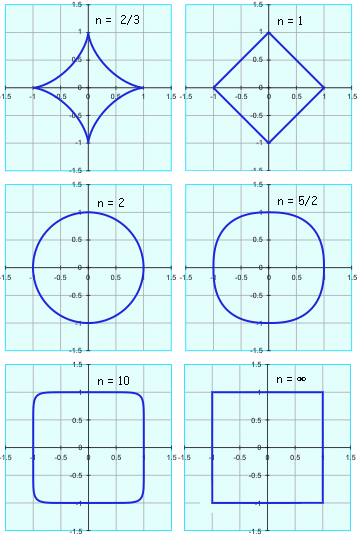All about flooble | fun stuff | Get a free chatterbox | Free JavaScript | Avatarsperplexus dot infoSquare the Circle (Posted on 2004-10-28)There is a family of curves on the Cartesian plane described by this form:If n is equal to 2, then it describes an ellipse (if a = b, then it describes a circle).

If n is greater than 2, then this is a "superellipse" (if a = b, then this is a supercircle).

As n increases, the ellipse becomes more "rectangularish", and as n approaches ∞, the limit is a rectangle (or a square if a=b).

What value must n have such that the figure has an area exactly halfway between the associated ellipse (when n=2) and rectangle (when n=∞)?

The graphs below, calculated by varying n with a = b = 1, show this property. Note that as n approaches zero, the curve degenerates into two crossed lines along the x- and y-axes.No Solution Yet Submitted by SilverKnight Rating: 3.0000 (4 votes)Comments: ( Back to comment list | You must be logged in to post comments.)sol using mathematica Comment 8 of 8 |FindRoot[Integrate[ (1 - x^n)^(1/n), {x, 0, 1}] == (Pi + 4)/8, {n, 1}]

generates solution
n = 3.16204
 Posted by sunny on 2004-10-31 11:57:12Please log in:

 Search: Search body:
Forums (1)